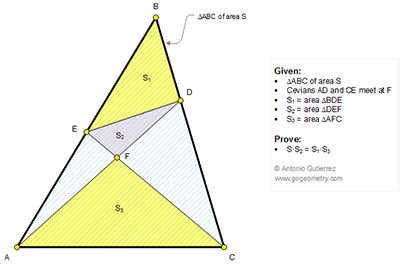## Thursday, July 9, 2015

### Geometry Problem 1131: Triangle Area, Two Cevians, Equal Product of Areas

Level: Mathematics Education, High School, Honors Geometry, College.

Click the diagram below to enlarge it.1.Let BF cut ED and AC at H and G
Observe that H and G are harmonic conjugate points of F and B
So GF/GB=HF/HB
But S3/S= GF/GB and S2/S3= HF/HB
The result will follow.

2.Lets compute the ratios S/S3 and S1/S2 and prove they are equal:

S3/ACD = AF/AD (triangles with same height)
ACD/S = CD/BC => ACD = (CD/BC)*S
S / S3 = (AD / AF) * (BC / CD)

ADE / S1 = AE / BE => ADE = (AE / BE) * S1
S1 / S2 = (AD / DF) * (BE / AE)

Next use Menelaus' theorem for the triangle ABD and the line CFE:

( BE / AE) * ( AF / DF ) * ( CD / BC ) = 1
( BE / AE) * ( AF / DF ) = BC / CD (multiply both sides by AD/AF)
( BE / AE) * ( AD / DF ) = (BC / CD) * (AD / AF)

These are exactly the expressions above for S1 / S2 and S / S3
Therefore S1 / S2 = S / S3

Hence S.S2 = S1.S3

3.From tr EDB and tr EDC => S1/(S2+S5) = BD/DC